Скачать презентацию PRICING FUTURES Factors that determine futures prices 1

befd3f0c357f4656db4f1691f11b74e0.ppt

• Количество слайдов: 28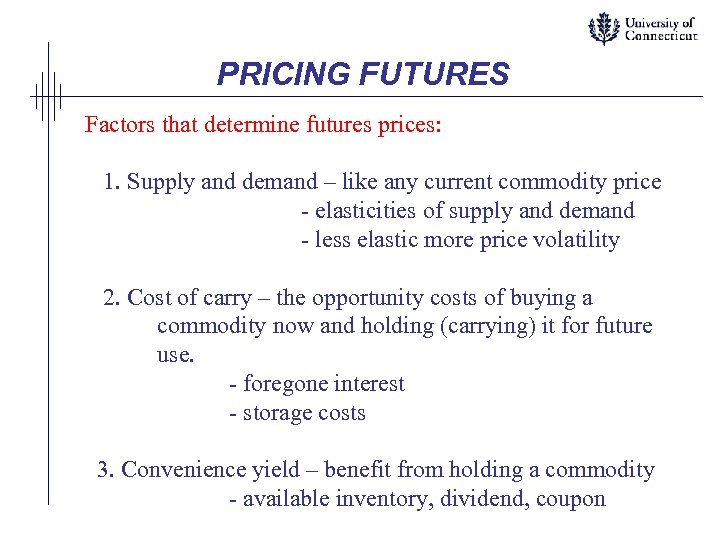PRICING FUTURES Factors that determine futures prices: 1. Supply and demand – like any current commodity price - elasticities of supply and demand - less elastic more price volatility 2. Cost of carry – the opportunity costs of buying a commodity now and holding (carrying) it for future use. - foregone interest - storage costs 3. Convenience yield – benefit from holding a commodity - available inventory, dividend, coupon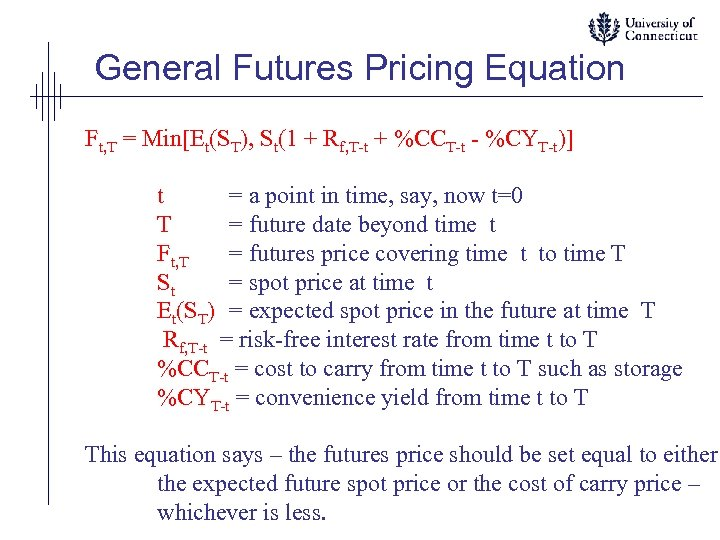General Futures Pricing Equation Ft, T = Min[Et(ST), St(1 + Rf, T-t + %CCT-t - %CYT-t)] t = a point in time, say, now t=0 T = future date beyond time t Ft, T = futures price covering time t to time T St = spot price at time t Et(ST) = expected spot price in the future at time T Rf, T-t = risk-free interest rate from time t to T %CCT-t = cost to carry from time t to T such as storage %CYT-t = convenience yield from time t to T This equation says – the futures price should be set equal to either the expected future spot price or the cost of carry price – whichever is less.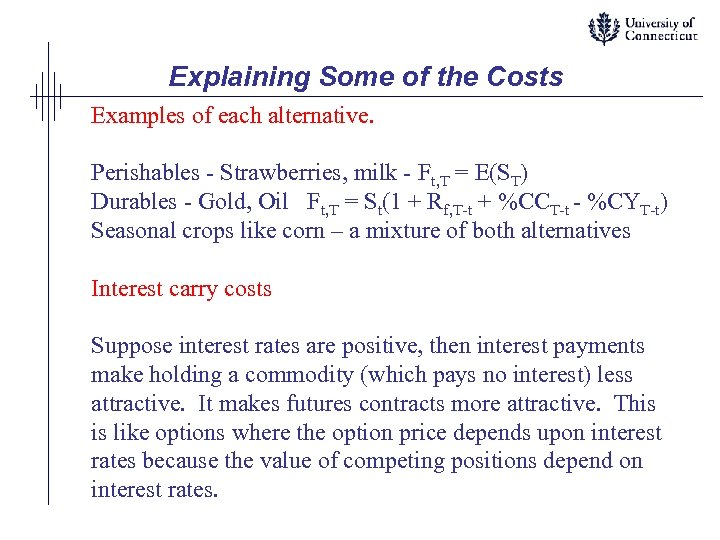Explaining Some of the Costs Examples of each alternative. Perishables - Strawberries, milk - Ft, T = E(ST) Durables - Gold, Oil Ft, T = St(1 + Rf, T-t + %CCT-t - %CYT-t) Seasonal crops like corn – a mixture of both alternatives Interest carry costs Suppose interest rates are positive, then interest payments make holding a commodity (which pays no interest) less attractive. It makes futures contracts more attractive. This is like options where the option price depends upon interest rates because the value of competing positions depend on interest rates.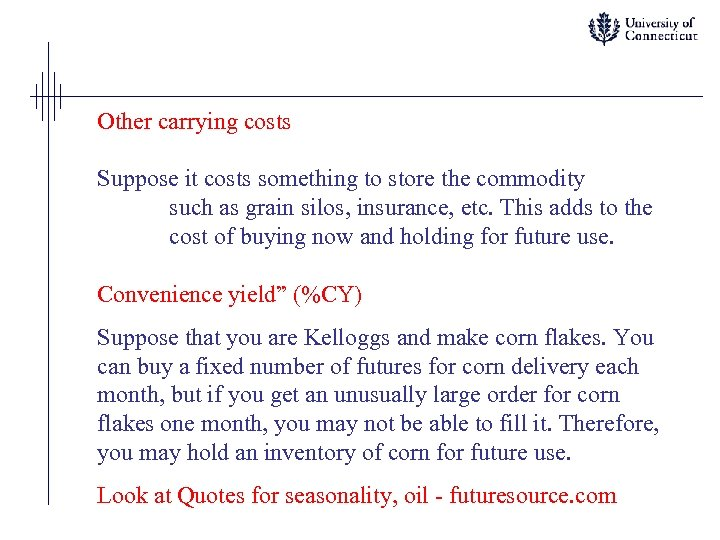Other carrying costs Suppose it costs something to store the commodity such as grain silos, insurance, etc. This adds to the cost of buying now and holding for future use. Convenience yield” (%CY) Suppose that you are Kelloggs and make corn flakes. You can buy a fixed number of futures for corn delivery each month, but if you get an unusually large order for corn flakes one month, you may not be able to fill it. Therefore, you may hold an inventory of corn for future use. Look at Quotes for seasonality, oil - futuresource. com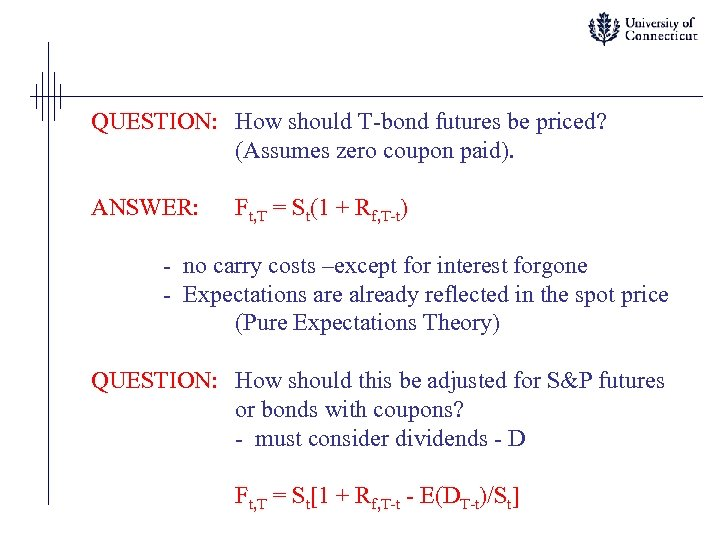QUESTION: How should T-bond futures be priced? (Assumes zero coupon paid). ANSWER: Ft, T = St(1 + Rf, T-t) - no carry costs –except for interest forgone - Expectations are already reflected in the spot price (Pure Expectations Theory) QUESTION: How should this be adjusted for S&P futures or bonds with coupons? - must consider dividends - D Ft, T = St[1 + Rf, T-t - E(DT-t)/St]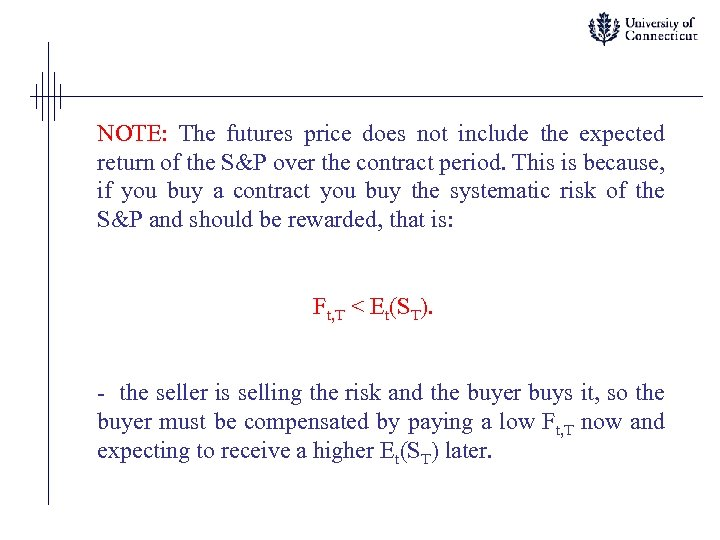NOTE: The futures price does not include the expected return of the S&P over the contract period. This is because, if you buy a contract you buy the systematic risk of the S&P and should be rewarded, that is: Ft, T < Et(ST). - the seller is selling the risk and the buyer buys it, so the buyer must be compensated by paying a low Ft, T now and expecting to receive a higher Et(ST) later.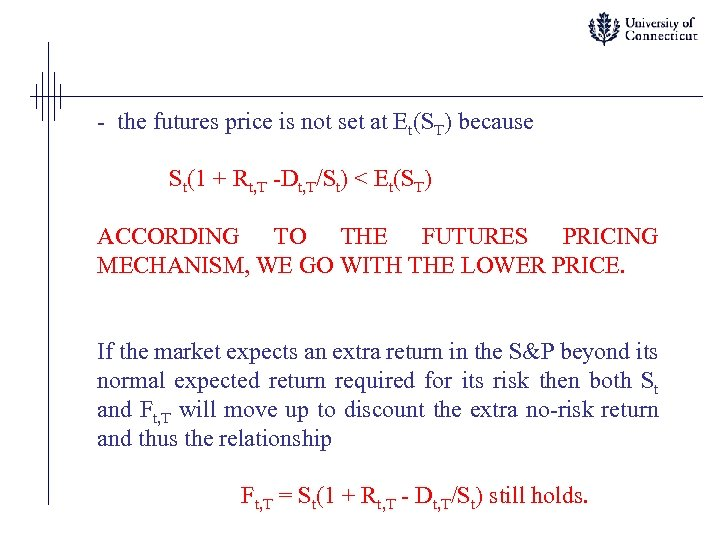- the futures price is not set at Et(ST) because St(1 + Rt, T -Dt, T/St) < Et(ST) ACCORDING TO THE FUTURES PRICING MECHANISM, WE GO WITH THE LOWER PRICE. If the market expects an extra return in the S&P beyond its normal expected return required for its risk then both St and Ft, T will move up to discount the extra no-risk return and thus the relationship Ft, T = St(1 + Rt, T - Dt, T/St) still holds.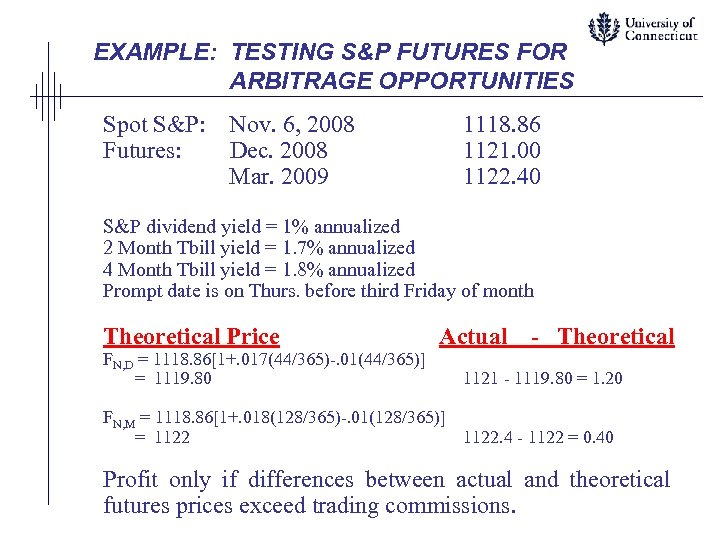EXAMPLE: TESTING S&P FUTURES FOR ARBITRAGE OPPORTUNITIES Spot S&P: Futures: Nov. 6, 2008 Dec. 2008 Mar. 2009 1118. 86 1121. 00 1122. 40 S&P dividend yield = 1% annualized 2 Month Tbill yield = 1. 7% annualized 4 Month Tbill yield = 1. 8% annualized Prompt date is on Thurs. before third Friday of month Theoretical Price FN, D = 1118. 86[1+. 017(44/365)-. 01(44/365)] = 1119. 80 Actual - Theoretical 1121 - 1119. 80 = 1. 20 FN, M = 1118. 86[1+. 018(128/365)-. 01(128/365)] = 1122. 4 - 1122 = 0. 40 Profit only if differences between actual and theoretical futures prices exceed trading commissions.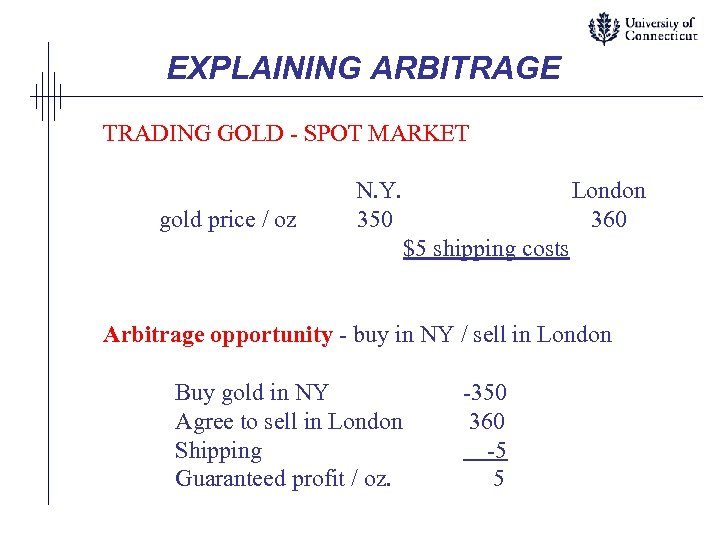EXPLAINING ARBITRAGE TRADING GOLD - SPOT MARKET gold price / oz N. Y. 350 London 360 \$5 shipping costs Arbitrage opportunity - buy in NY / sell in London Buy gold in NY Agree to sell in London Shipping Guaranteed profit / oz. -350 360 -5 5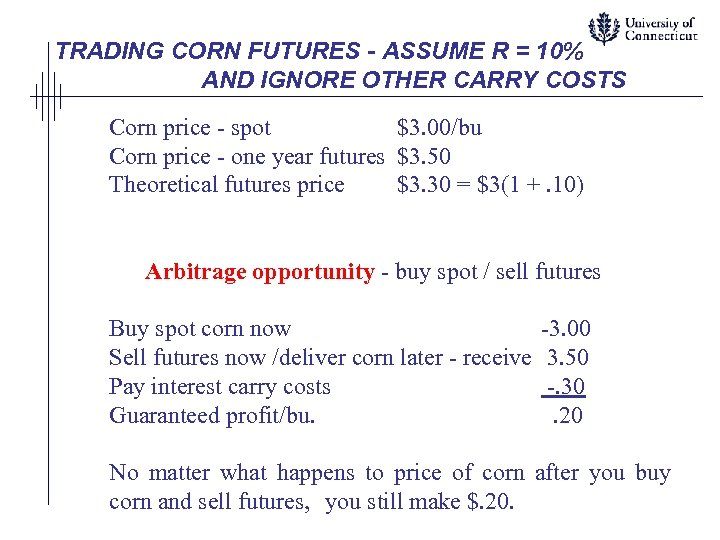TRADING CORN FUTURES - ASSUME R = 10% AND IGNORE OTHER CARRY COSTS Corn price - spot \$3. 00/bu Corn price - one year futures \$3. 50 Theoretical futures price \$3. 30 = \$3(1 +. 10) Arbitrage opportunity - buy spot / sell futures Buy spot corn now Sell futures now /deliver corn later - receive Pay interest carry costs Guaranteed profit/bu. -3. 00 3. 50 -. 30. 20 No matter what happens to price of corn after you buy corn and sell futures, you still make \$. 20.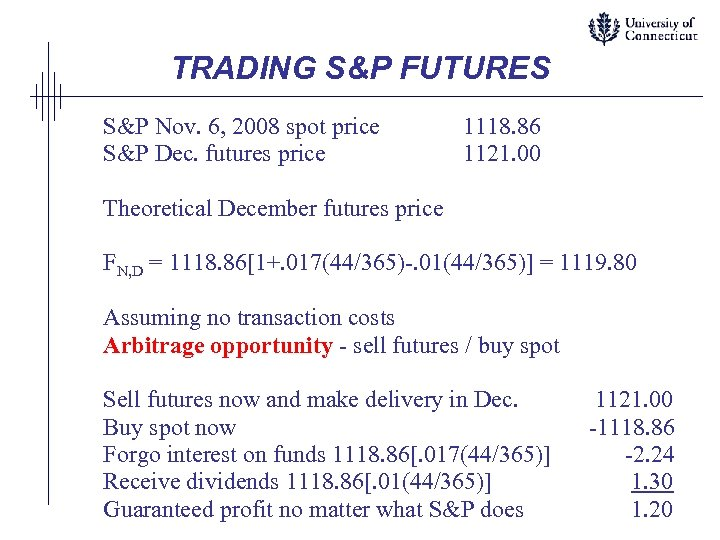TRADING S&P FUTURES S&P Nov. 6, 2008 spot price S&P Dec. futures price 1118. 86 1121. 00 Theoretical December futures price FN, D = 1118. 86[1+. 017(44/365)-. 01(44/365)] = 1119. 80 Assuming no transaction costs Arbitrage opportunity - sell futures / buy spot Sell futures now and make delivery in Dec. Buy spot now Forgo interest on funds 1118. 86[. 017(44/365)] Receive dividends 1118. 86[. 01(44/365)] Guaranteed profit no matter what S&P does 1121. 00 -1118. 86 -2. 24 1. 30 1. 20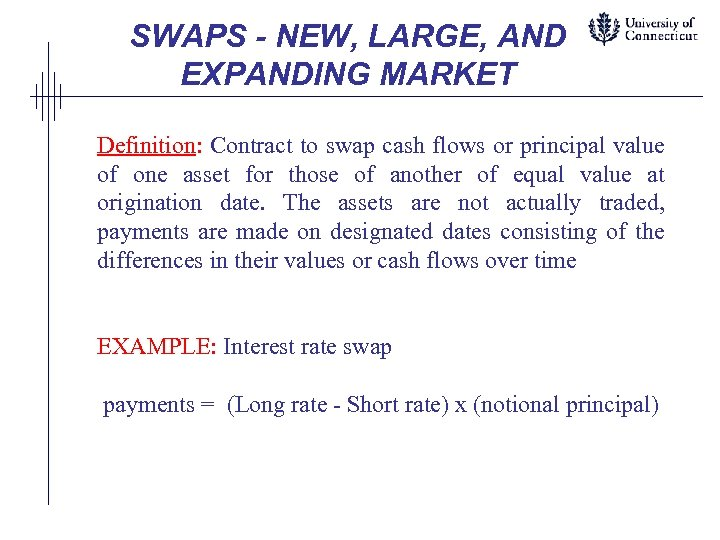SWAPS - NEW, LARGE, AND EXPANDING MARKET Definition: Contract to swap cash flows or principal value of one asset for those of another of equal value at origination date. The assets are not actually traded, payments are made on designated dates consisting of the differences in their values or cash flows over time EXAMPLE: Interest rate swap payments = (Long rate - Short rate) x (notional principal)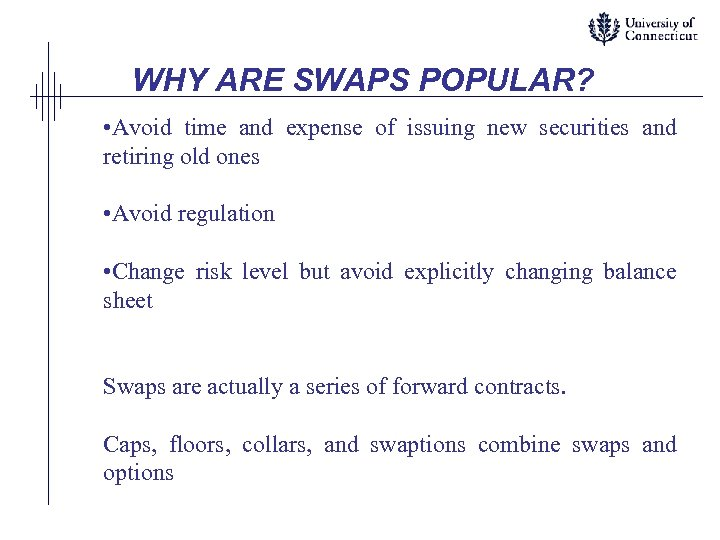WHY ARE SWAPS POPULAR? • Avoid time and expense of issuing new securities and retiring old ones • Avoid regulation • Change risk level but avoid explicitly changing balance sheet Swaps are actually a series of forward contracts. Caps, floors, collars, and swaptions combine swaps and options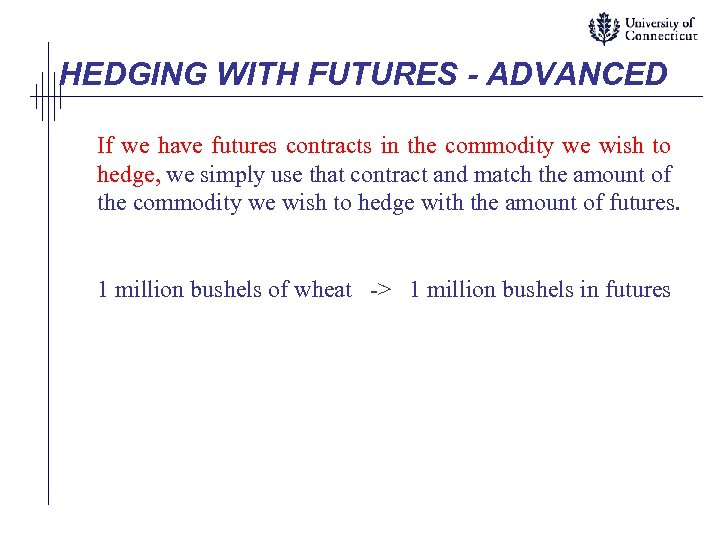HEDGING WITH FUTURES - ADVANCED If we have futures contracts in the commodity we wish to hedge, we simply use that contract and match the amount of the commodity we wish to hedge with the amount of futures. 1 million bushels of wheat -> 1 million bushels in futures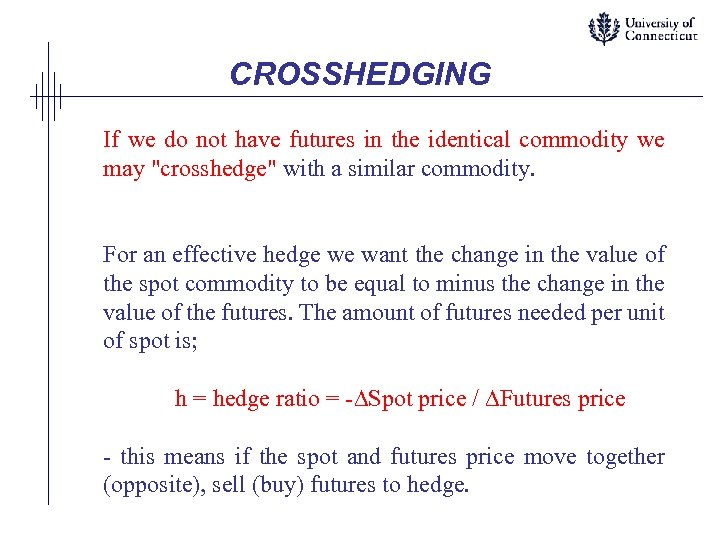CROSSHEDGING If we do not have futures in the identical commodity we may "crosshedge" with a similar commodity. For an effective hedge we want the change in the value of the spot commodity to be equal to minus the change in the value of the futures. The amount of futures needed per unit of spot is; h = hedge ratio = -DSpot price / DFutures price - this means if the spot and futures price move together (opposite), sell (buy) futures to hedge.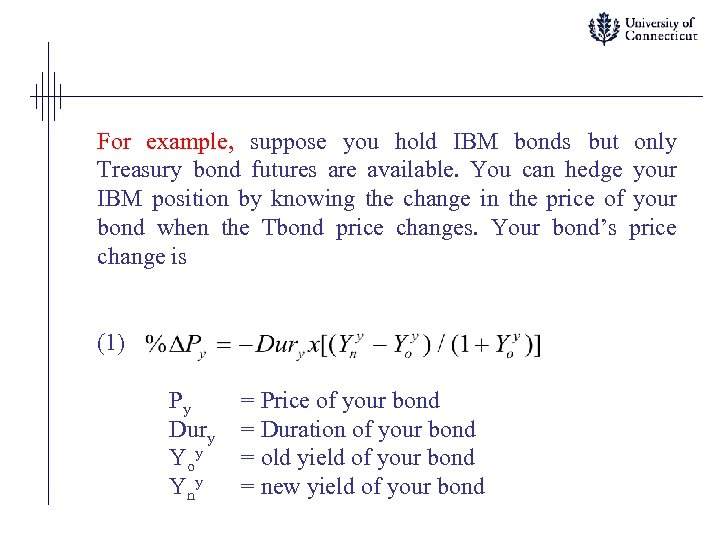For example, suppose you hold IBM bonds but only Treasury bond futures are available. You can hedge your IBM position by knowing the change in the price of your bond when the Tbond price changes. Your bond’s price change is (1) Py Dury Yoy Yny = Price of your bond = Duration of your bond = old yield of your bond = new yield of your bond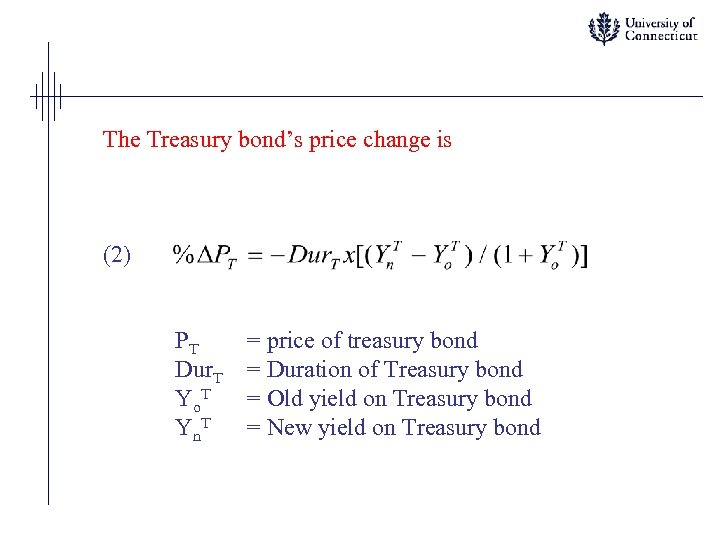The Treasury bond’s price change is (2) PT Dur. T Yo. T Yn. T = price of treasury bond = Duration of Treasury bond = Old yield on Treasury bond = New yield on Treasury bond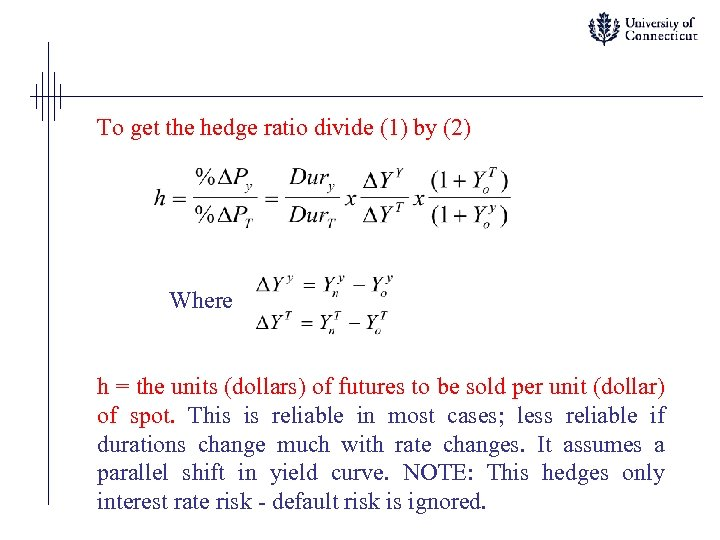To get the hedge ratio divide (1) by (2) Where h = the units (dollars) of futures to be sold per unit (dollar) of spot. This is reliable in most cases; less reliable if durations change much with rate changes. It assumes a parallel shift in yield curve. NOTE: This hedges only interest rate risk - default risk is ignored.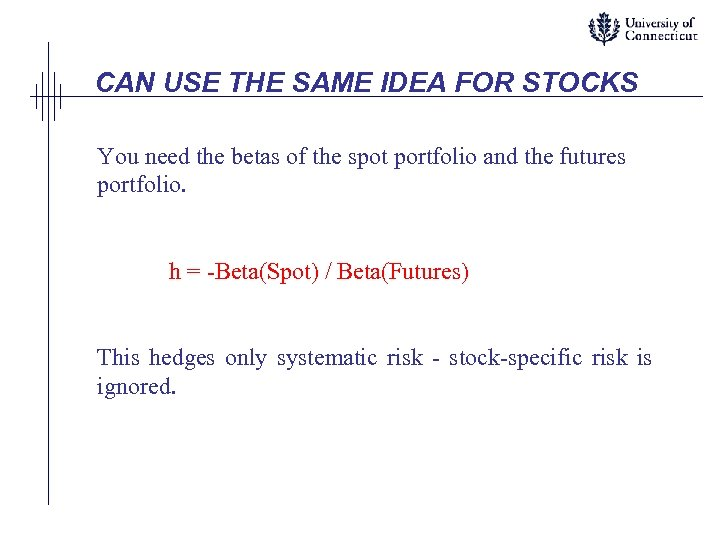CAN USE THE SAME IDEA FOR STOCKS You need the betas of the spot portfolio and the futures portfolio. h = -Beta(Spot) / Beta(Futures) This hedges only systematic risk - stock-specific risk is ignored.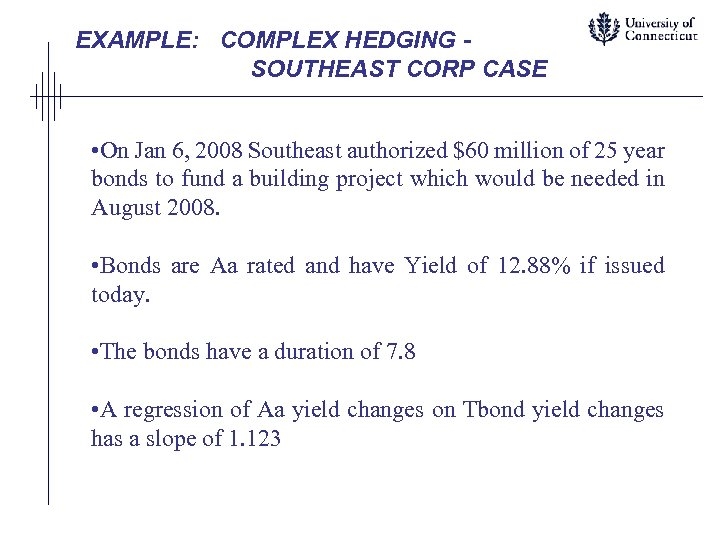EXAMPLE: COMPLEX HEDGING SOUTHEAST CORP CASE • On Jan 6, 2008 Southeast authorized \$60 million of 25 year bonds to fund a building project which would be needed in August 2008. • Bonds are Aa rated and have Yield of 12. 88% if issued today. • The bonds have a duration of 7. 8 • A regression of Aa yield changes on Tbond yield changes has a slope of 1. 123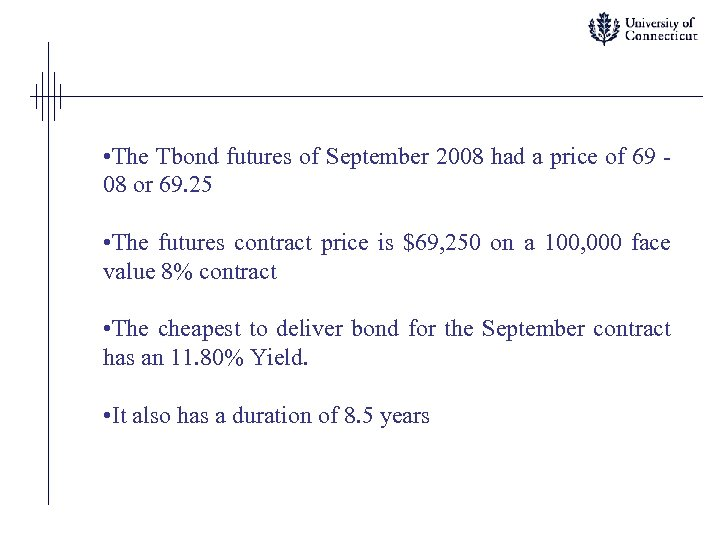• The Tbond futures of September 2008 had a price of 69 08 or 69. 25 • The futures contract price is \$69, 250 on a 100, 000 face value 8% contract • The cheapest to deliver bond for the September contract has an 11. 80% Yield. • It also has a duration of 8. 5 years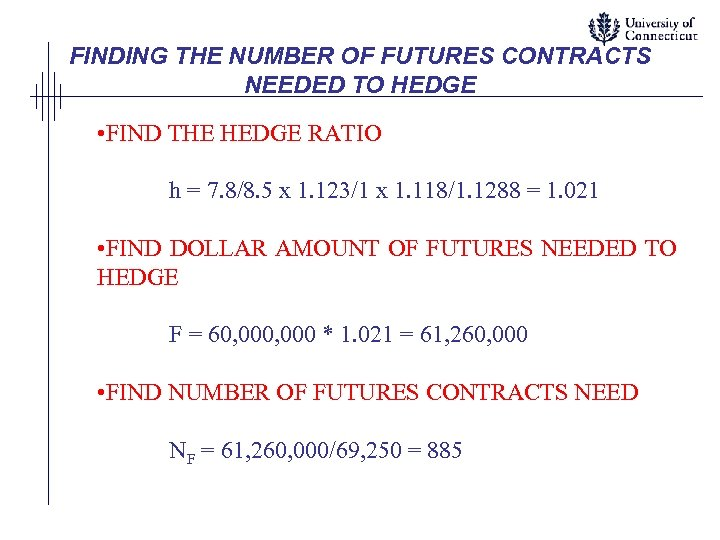FINDING THE NUMBER OF FUTURES CONTRACTS NEEDED TO HEDGE • FIND THE HEDGE RATIO h = 7. 8/8. 5 x 1. 123/1 x 1. 118/1. 1288 = 1. 021 • FIND DOLLAR AMOUNT OF FUTURES NEEDED TO HEDGE F = 60, 000 * 1. 021 = 61, 260, 000 • FIND NUMBER OF FUTURES CONTRACTS NEED NF = 61, 260, 000/69, 250 = 885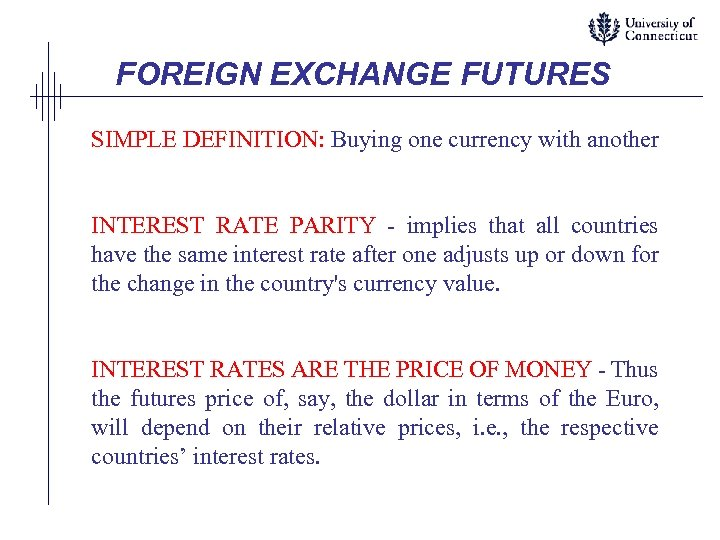FOREIGN EXCHANGE FUTURES SIMPLE DEFINITION: Buying one currency with another INTEREST RATE PARITY - implies that all countries have the same interest rate after one adjusts up or down for the change in the country's currency value. INTEREST RATES ARE THE PRICE OF MONEY - Thus the futures price of, say, the dollar in terms of the Euro, will depend on their relative prices, i. e. , the respective countries’ interest rates.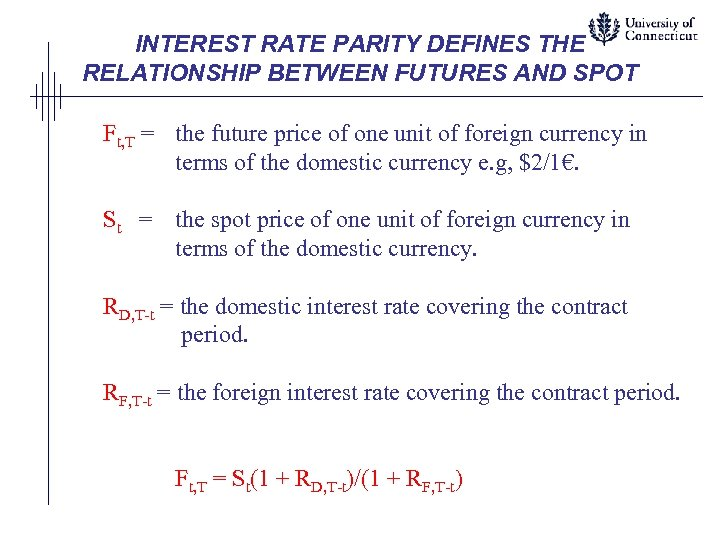INTEREST RATE PARITY DEFINES THE RELATIONSHIP BETWEEN FUTURES AND SPOT Ft, T = the future price of one unit of foreign currency in terms of the domestic currency e. g, \$2/1€. St = the spot price of one unit of foreign currency in terms of the domestic currency. RD, T-t = the domestic interest rate covering the contract period. RF, T-t = the foreign interest rate covering the contract period. Ft, T = St(1 + RD, T-t)/(1 + RF, T-t)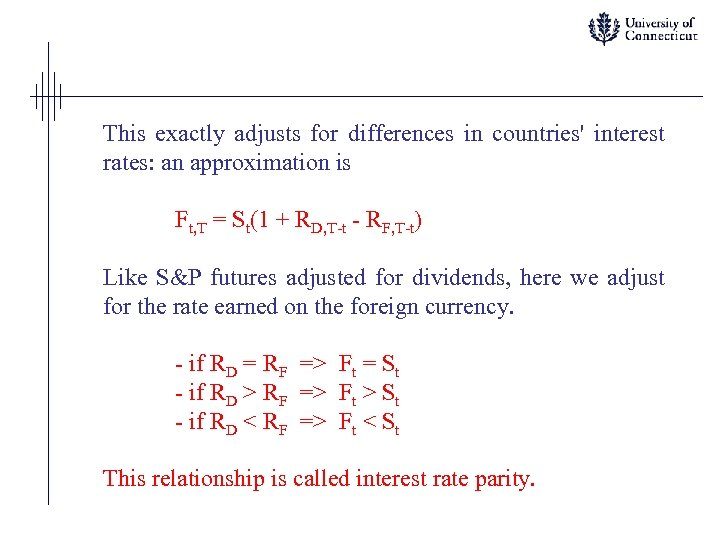This exactly adjusts for differences in countries' interest rates: an approximation is Ft, T = St(1 + RD, T-t - RF, T-t) Like S&P futures adjusted for dividends, here we adjust for the rate earned on the foreign currency. - if RD = RF => Ft = St - if RD > RF => Ft > St - if RD < RF => Ft < St This relationship is called interest rate parity.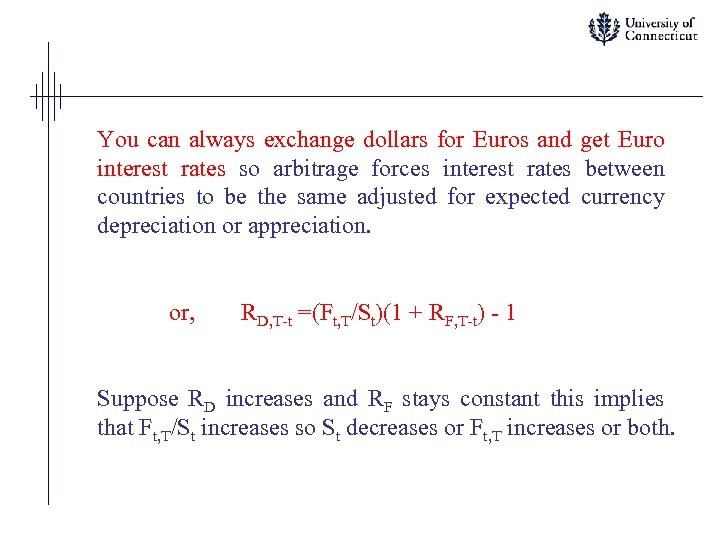You can always exchange dollars for Euros and get Euro interest rates so arbitrage forces interest rates between countries to be the same adjusted for expected currency depreciation or appreciation. or, RD, T-t =(Ft, T/St)(1 + RF, T-t) - 1 Suppose RD increases and RF stays constant this implies that Ft, T/St increases so St decreases or Ft, T increases or both.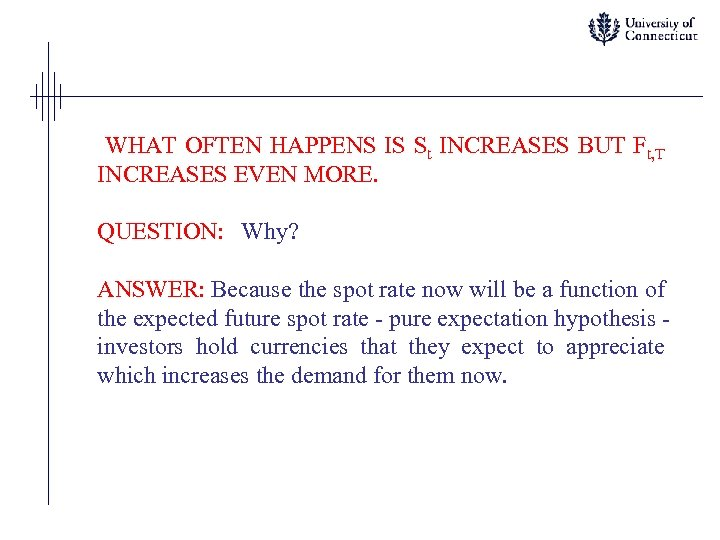WHAT OFTEN HAPPENS IS St INCREASES BUT Ft, T INCREASES EVEN MORE. QUESTION: Why? ANSWER: Because the spot rate now will be a function of the expected future spot rate - pure expectation hypothesis investors hold currencies that they expect to appreciate which increases the demand for them now.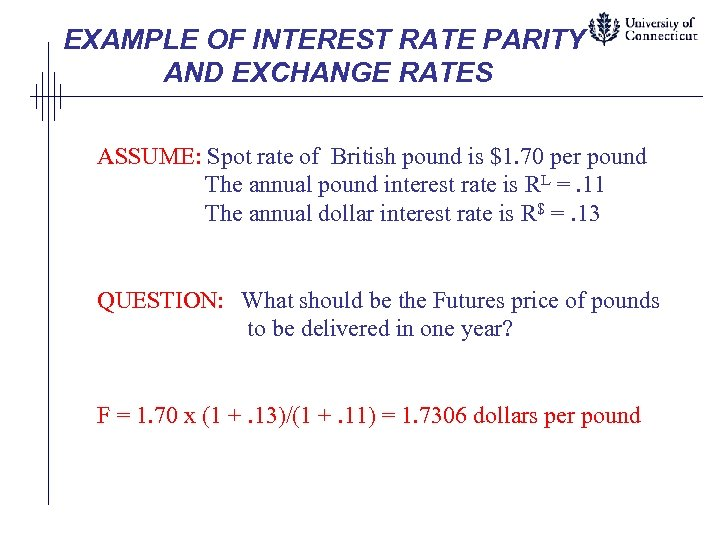EXAMPLE OF INTEREST RATE PARITY AND EXCHANGE RATES ASSUME: Spot rate of British pound is \$1. 70 per pound The annual pound interest rate is RL =. 11 The annual dollar interest rate is R\$ =. 13 QUESTION: What should be the Futures price of pounds to be delivered in one year? F = 1. 70 x (1 +. 13)/(1 +. 11) = 1. 7306 dollars per pound